# sklearn.utils.shuffle¶

sklearn.utils.shuffle(*arrays, random_state=None, n_samples=None)[source]

Shuffle arrays or sparse matrices in a consistent way.

This is a convenience alias to resample(*arrays, replace=False) to do random permutations of the collections.

Parameters:
*arrayssequence of indexable data-structures

Indexable data-structures can be arrays, lists, dataframes or scipy sparse matrices with consistent first dimension.

random_stateint, RandomState instance or None, default=None

Determines random number generation for shuffling the data. Pass an int for reproducible results across multiple function calls. See Glossary.

n_samplesint, default=None

Number of samples to generate. If left to None this is automatically set to the first dimension of the arrays. It should not be larger than the length of arrays.

Returns:
shuffled_arrayssequence of indexable data-structures

Sequence of shuffled copies of the collections. The original arrays are not impacted.

resample

Resample arrays or sparse matrices in a consistent way.

Examples

It is possible to mix sparse and dense arrays in the same run:

>>> import numpy as np
>>> X = np.array([[1., 0.], [2., 1.], [0., 0.]])
>>> y = np.array([0, 1, 2])

>>> from scipy.sparse import coo_matrix
>>> X_sparse = coo_matrix(X)

>>> from sklearn.utils import shuffle
>>> X, X_sparse, y = shuffle(X, X_sparse, y, random_state=0)
>>> X
array([[0., 0.],
[2., 1.],
[1., 0.]])

>>> X_sparse
<3x2 sparse matrix of type '<... 'numpy.float64'>'
with 3 stored elements in Compressed Sparse Row format>

>>> X_sparse.toarray()
array([[0., 0.],
[2., 1.],
[1., 0.]])

>>> y
array([2, 1, 0])

>>> shuffle(y, n_samples=2, random_state=0)
array([0, 1])


## Examples using sklearn.utils.shuffle¶Color Quantization using K-Means

Color Quantization using K-Means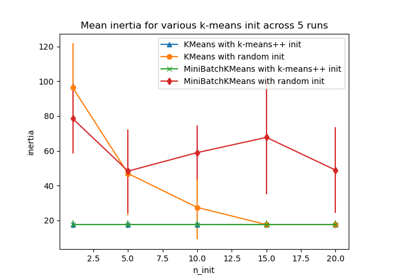Empirical evaluation of the impact of k-means initialization

Empirical evaluation of the impact of k-means initialization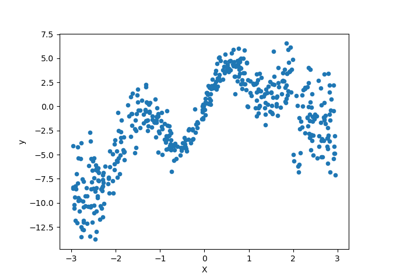Combine predictors using stacking

Combine predictors using stacking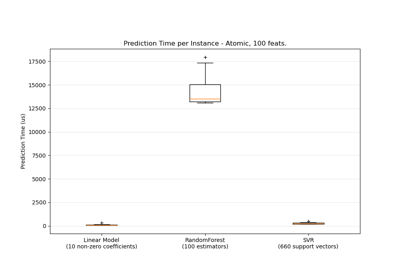Prediction Latency

Prediction Latency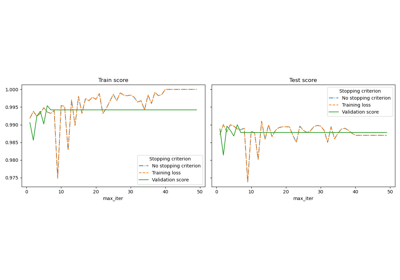Early stopping of Stochastic Gradient Descent

Early stopping of Stochastic Gradient DescentApproximate nearest neighbors in TSNE

Approximate nearest neighbors in TSNE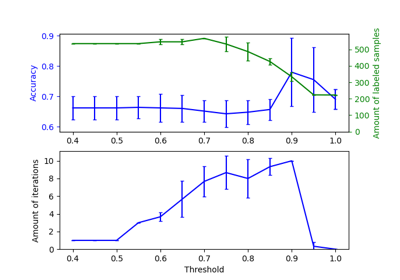Effect of varying threshold for self-training

Effect of varying threshold for self-training